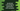# JavaScript reduceRight() method explanation with examples## JavaScript reduceRight() method explanation with examples:

The reduceRight method can be used to get a single result by applying an accumulator from right to left of an array. In this post, we will learn how to use reduceRight method with different examples.

### Syntax of reduceRight:

We can use an array function, a callback function or a callback reducer function. It is defined as like below:

#### With arrow functions:

``````reduceRight((acc, v) => {});
reduceRight((acc, v, i) => {});
reduceRight((acc, v, i, arr) => {});
reduceRight((acc, v, i, arr) => {}, startValue);``````

#### With callback function:

``````reduceRight(fun);
reduceRight(fun, startValue);``````

#### With callback reducer functions:

``````reduceRight(function(acc, v){});
reduceRight(function(acc, v, i){});
reduceRight(function(acc, v, i, arr){});
reduceRight(function(acc, v, i, arr){}, startValue);``````

Here,

• acc is the accumulator value. It is the value returned in the last iteration. This value will be the final value once the iteration is completed.

• v is the current processing value.

• i is an optional value. It is the index of the current element being processed.

• arr is also an optional value. It is the array, i.e. where we are calling reduceRight.

• startValue is another optional value. It is the accumulator value to the first call of the callback function.

• Note that if we don’t provide the initial value, it will pick the last element of the array. If we call it on an empty array without the initial value, it will throw a TypeError.

### Return value of reduceRight:

It will return the final result value.

### Example of reduceRight:

Let’s take an example of reduceRight:

``````const arr = [1, 2, 3, 4];

let result = arr.reduceRight((acc, v, i, arr) => {
return acc + v;
});

console.log(result);``````

In this example,

• It is using reduceRight to find the sum of all numbers in the array arr.
• We are using an arrow function inside reduceRight. It returns the sum of current value and the value of accumulator.

If we check for the above example, it will work as like below:

step acc v i arr
1st 4 3 2 [1,2,3,4]
2nd 7 2 1 [1,2,3,4]
3rd 9 1 0 [1,2,3,4]

You can see that, since we are not passing any initial value to the accumulator, it picked the rightmost element and it is starting from the second right.

The index it iterates from right to left.

It will print 10, i.e. the sum of all the numbers in that array.

### Example of reduceRight with start value:

Let’s try reduceRight with a start value:

``````const arr = [1, 2, 3, 4];

let result = arr.reduceRight((acc, v) => {
return acc + v;
}, 10);

console.log(result);``````

It is the same program that we discussed before. The only difference is that we are passing 10 as the start value.

It will work as like below:

step acc v i arr
1st 10 4 3 [1,2,3,4]
2nd 14 3 2 [1,2,3,4]
3rd 17 2 1 [1,2,3,4]
4th 19 1 0 [1,2,3,4]

So, it starts from the last value, i.e. the number at index 3 and the accumulator value is 10 for the first iteration. For the next iterations, it keeps adding the current value of the array. It will print 19.

### Example of reduceRight with an empty array:

Let’s try with an empty array with a start value:

``````const arr = [];

let result = arr.reduceRight((acc, v) => {
return acc + v;
}, 10);

console.log(result);``````

Here, arr is an empty array and we are passing 10 as the starting value. If you run it, it will print 10 since the array is empty.

But, if we don’t provide the start value:

``````const arr = [];

let result = arr.reduceRight((acc, v) => {
return acc + v;
});

console.log(result);``````

It will throw a TypeError.